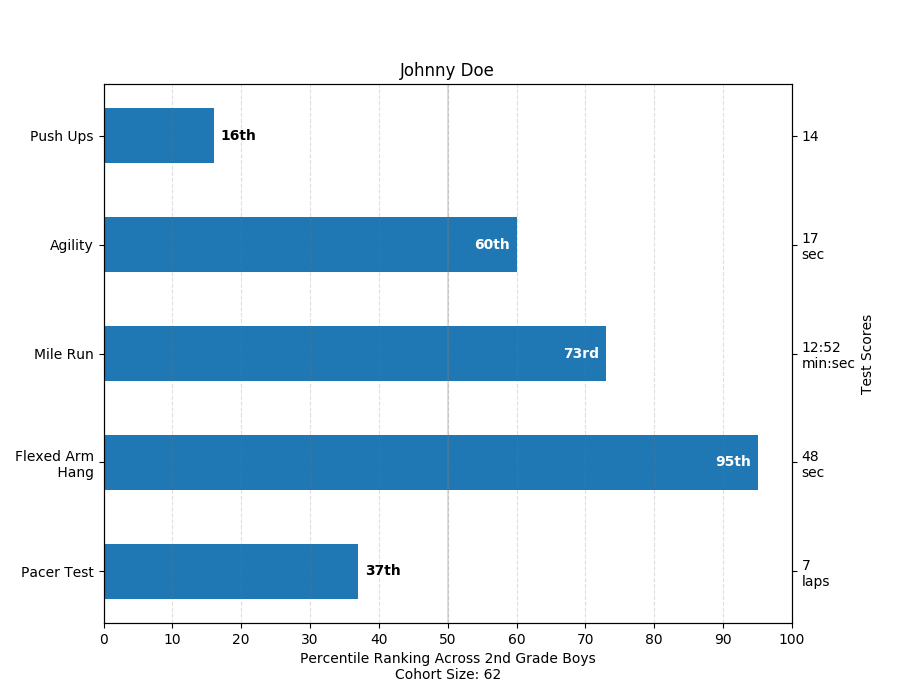# Percentiles as horizontal bar chart¶

Bar charts are useful for visualizing counts, or summary statistics with error bars. Also see the Grouped bar chart with labels or the Horizontal bar chart example for simpler versions of those features.

This example comes from an application in which grade school gym teachers wanted to be able to show parents how their child did across a handful of fitness tests, and importantly, relative to how other children did. To extract the plotting code for demo purposes, we'll just make up some data for little Johnny Doe.

import numpy as np
import matplotlib
import matplotlib.pyplot as plt
from matplotlib.ticker import MaxNLocator
from collections import namedtuple

np.random.seed(42)

Student = namedtuple('Student', ['name', 'grade', 'gender'])
Score = namedtuple('Score', ['score', 'percentile'])

# GLOBAL CONSTANTS
testNames = ['Pacer Test', 'Flexed Arm\n Hang', 'Mile Run', 'Agility',
'Push Ups']
testMeta = dict(zip(testNames, ['laps', 'sec', 'min:sec', 'sec', '']))

def attach_ordinal(num):
"""helper function to add ordinal string to integers

1 -> 1st
56 -> 56th
"""
suffixes = {str(i): v
for i, v in enumerate(['th', 'st', 'nd', 'rd', 'th',
'th', 'th', 'th', 'th', 'th'])}

v = str(num)
# special case early teens
if v in {'11', '12', '13'}:
return v + 'th'
return v + suffixes[v[-1]]

def format_score(scr, test):
"""
Build up the score labels for the right Y-axis by first
the appropriate meta information (i.e., 'laps' vs 'seconds'). We
want the labels centered on the ticks, so if there is no meta
the string
"""
md = testMeta[test]
if md:
return '{0}\n{1}'.format(scr, md)
else:
return scr

def format_ycursor(y):
y = int(y)
if y < 0 or y >= len(testNames):
return ''
else:
return testNames[y]

def plot_student_results(student, scores, cohort_size):
#  create the figure
fig, ax1 = plt.subplots(figsize=(9, 7))

pos = np.arange(len(testNames))

rects = ax1.barh(pos, [scores[k].percentile for k in testNames],
align='center',
height=0.5,
tick_label=testNames)

ax1.set_title(student.name)

ax1.set_xlim([0, 100])
ax1.xaxis.set_major_locator(MaxNLocator(11))
ax1.xaxis.grid(True, linestyle='--', which='major',
color='grey', alpha=.25)

# Plot a solid vertical gridline to highlight the median position
ax1.axvline(50, color='grey', alpha=0.25)

# Set the right-hand Y-axis ticks and labels
ax2 = ax1.twinx()

scoreLabels = [format_score(scores[k].score, k) for k in testNames]

# set the tick locations
ax2.set_yticks(pos)
# make sure that the limits are set equally on both yaxis so the
# ticks line up
ax2.set_ylim(ax1.get_ylim())

# set the tick labels
ax2.set_yticklabels(scoreLabels)

ax2.set_ylabel('Test Scores')

'Cohort Size: {cohort_size}')
gender=student.gender.title(),
cohort_size=cohort_size))

rect_labels = []
# Lastly, write in the ranking inside each bar to aid in interpretation
for rect in rects:
# Rectangle widths are already integer-valued but are floating
# type, so it helps to remove the trailing decimal point and 0 by
# converting width to int type
width = int(rect.get_width())

rankStr = attach_ordinal(width)
# The bars aren't wide enough to print the ranking inside
if width < 40:
# Shift the text to the right side of the right edge
xloc = 5
# Black against white background
clr = 'black'
align = 'left'
else:
# Shift the text to the left side of the right edge
xloc = -5
# White on magenta
clr = 'white'
align = 'right'

# Center the text vertically in the bar
yloc = rect.get_y() + rect.get_height() / 2
label = ax1.annotate(rankStr, xy=(width, yloc), xytext=(xloc, 0),
textcoords="offset points",
ha=align, va='center',
color=clr, weight='bold', clip_on=True)
rect_labels.append(label)

# make the interactive mouse over give the bar title
ax2.fmt_ydata = format_ycursor
# return all of the artists created
return {'fig': fig,
'ax': ax1,
'ax_right': ax2,
'bars': rects,
'perc_labels': rect_labels}

student = Student('Johnny Doe', 2, 'boy')
scores = dict(zip(testNames,
(Score(v, p) for v, p in
zip(['7', '48', '12:52', '17', '14'],
np.round(np.random.uniform(0, 1,
len(testNames)) * 100, 0)))))
cohort_size = 62  # The number of other 2nd grade boys

arts = plot_student_results(student, scores, cohort_size)
plt.show()